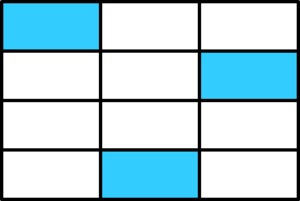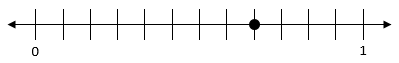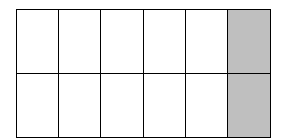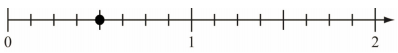# Fraction Equivalence and Ordering

## Objective

Recognize and generate equivalent fractions with larger units using visual models.

## Common Core Standards

### Core Standards

?

• 4.NF.A.1 — Explain why a fraction a/b is equivalent to a fraction (n × a)/(n × b) by using visual fraction models, with attention to how the number and size of the parts differ even though the two fractions themselves are the same size. Use this principle to recognize and generate equivalent fractions.

?

• 3.NF.A.2

• 4.OA.A.1

• 4.OA.A.2

## Criteria for Success

?

1. Understand that equivalent fractions are fractions that refer to the same whole and are the same size.
2. Generate equivalent fractions with larger units using visual models (MP.5).
3. Generate equivalent fractions with larger units when given either the number of units (the numerator) or the size of the unit (the denominator) in the equivalent fraction.
4. Show that two fractions are equivalent using visual models and explain why the models demonstrate their equivalence (MP.3).

## Tips for Teachers

?

• After spending lots of time exploring various fraction models and how to use them to recognize and generate larger equivalent fractions, students will work with all models in this lesson. All three are included in this lesson since students should feel much more comfortable with their use after going so in-depth in Lessons 1–3 and so that they can progress to the general method involving division more quickly since they’re likely able to deduce it fairly quickly after Lesson 4. However, if students seem to need more work with any model in particular, you may choose to break this into two lessons and focus exclusively on that model in need of more work on the additional day.
• As a supplement to the Problem Set, students can play a game to practice finding equivalent fractions with larger units using visual models, such as “Show Me 2” from Games with Fraction Strips and Fraction Cards on The Max Ray Blog.

#### Fishtank Plus

• Problem Set
• Student Handout Editor
• Vocabulary Package

?

### Problem 1

a. What fraction of the rectangle below is shaded?b. Laura says that ${{1\over4}}$ of the model is shaded. Do you think she is correct? Explain why or why not by using a picture.

#### References

Illustrative Mathematics Fractions and Rectangles

Fractions and Rectangles, accessed on March 23, 2018, 9:23 a.m., is licensed by Illustrative Mathematics under either the CC BY 4.0 or CC BY-NC-SA 4.0. For further information, contact Illustrative Mathematics.

### Problem 2

A point is shown on the number line diagram below.Write three equivalent fractions that name this point.

#### References

Achieve the Core Fraction Concepts Mini-AssessmentQuestion #2

Fraction Concepts Mini-Assessment by Student Achievement Partners is made available by Achieve the Core under the CC0 1.0 license. Accessed March 23, 2018, 9:16 a.m..

Modified by The Match Foundation, Inc.

### Problem 3

Solve for the missing value.

a.     ${{20\over24}={\square\over6}}$

b.     ${{32\over10}={16\over\square}}$

## Problem Set & Homework

#### Discussion of Problem Set

• Look at #2c. What denominator made this statement true? Is there another way to rename this fraction? (Discuss how this is equivalent to the whole number 2.)
• How did you shade the fraction strip in #3? What made this pair of equivalent fractions different from many of the others we’ve looked at so far? Because of that difference, how could you ensure that they were equivalent?
• Look at #4. I think the correct answer is C. Do you agree or disagree? Why? What error did I make?

?

### Problem 1

Ryan folded a sheet of paper to make 12 equal-sized sections. He shaded 2 sections, as shown below.Which fraction is equivalent to the one represented by the shaded part of the sheet of paper?

a     ${{1\over12}}$

b.     ${{1\over6}}$

c.     ${{1\over5}}$

d.     ${{6\over5}}$

#### References

Question #3

From EngageNY.org of the New York State Education Department. New York State Testing Program Grade 4 Common Core Mathematics Test Released Questions July 2015. Internet. Available from https://www.engageny.org/resource/released-2015-3-8-ela-and-mathematics-state-test-questions/file/132976; accessed March 10, 2017, 4:34 p.m..

### Problem 2

A point is shown on the number line diagram below.Write three equivalent fractions that name this point.

#### References

Achieve the Core Fraction Concepts Mini-AssessmentQuestion #1

Fraction Concepts Mini-Assessment by Student Achievement Partners is made available by Achieve the Core under the CC0 1.0 license. Accessed March 23, 2018, 9:16 a.m..

### Problem 3

Use the number line to explain how you know the fractions in #2 are equivalent.

?